Year 3 Objectives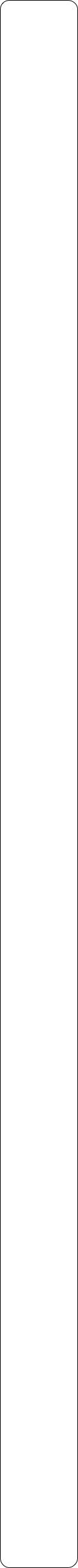Place ValuePre Tasks

(PitStops)

Main Tasks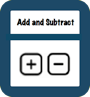Pre Tasks

(PitStops)

Main Tasks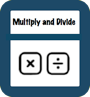Multiplication and Division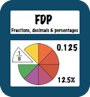Pre Tasks

(PitStops)

Main Tasks

Pre Tasks

(PitStops)

Main Tasks

Number - Fractions

Addition and Subtraction1. -Count from 0 in multiples of 4, 8, 50 and 100

1. -Find 10 or 100 more or less than a given number

- Recognise the place value of each digit in a three-digit number (hundreds, tens, ones)

- Compare and order numbers up to 1000

- Identify, represent and estimate numbers using different representations

- Read and write numbers up to 1000 in numerals and in words

- Solve number problems and practical problems involving these ideas- a three-digit number and ones

- a three-digit number and tens

- a three-digit number and hundreds

- Add and subtract numbers with up to three digits, using formal written methods of columnar addition and subtraction

- Estimate the answer to a calculation and use inverse operations to check answers

- Solve problems, including missing number problems, using number facts, place value, and more complex addition and subtraction

Add and subtract numbers mentally, including:- Recall and use multiplication and division facts for the x3, x4 and x8 multiplication tables

- Write and calculate mathematical statements for multiplication and division using the multiplication tables that they know, including for two-digit numbers times one-digit numbers, using mental and progressing to formal written methods

- Solve problems, including missing number problems, involving multiplication and division, including positive integer scaling problems and correspondence problems in which n objects are connected to m objects.- Count up and down in tenths; recognise that tenths arise from dividing an object into 10 equal parts and in dividing one-digit numbers or quantities by 10

- Recognise, find and write fractions of a discrete set of objects: unit fractions and non-unit fractions with small denominators

- Recognise and use fractions as numbers: unit fractions and non-unit fractions with small denominators

- Recognise and show, using diagrams, equivalent fractions with small denominators

- Add and subtract fractions with the same denominator within one whole [for example, 5/7 + 1/7=6/7 ]

- Compare and order unit fractions, and fractions with the same denominators

- Solve problems that involve all of the above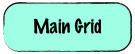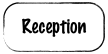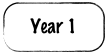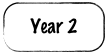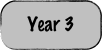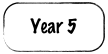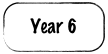Solutions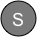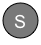StopsGap

StopsGap

StopsGap

StopsGap